Original Source

..

Theory of Everything

Doctor James Schombert
Lecture #17, May 5, 2004
Physics Department
University of Oregon

..
Quantum Electrodynamics :

 the combination of light and charged particles understood through quantum electrodynamics central to QED is the idea that virtual photons carry electromagnetic force however, virtual means they cannot be seen or detected because their existence violates conservation laws The subfield of physics that explains the interaction of charged particles and light is called quantum electrodynamics. Quantum electrodynamics (QED) extends quantum theory to fields of force, starting with electromagnetic fields. .. Quantum electrodynamics, or QED, is a quantum theory of the interactions of charged particles with the electromagnetic field. It describes mathematically not only all interactions of light with matter but also those of charged particles with one another. QED is a relativistic theory in that Albert Einstein's theory of special relativity is built into each of its equations. Because the behavior of atoms and molecules is primarily electromagnetic in nature, all of atomic physics can be considered a test laboratory for the theory. Agreement of such high accuracy makes QED one of the most successful physical theories so far devised. .. In 1926 the British physicist P.A.M. Dirac laid the foundations for QED with his discovery of an equation describing the motion and spin of electrons that incorporated both the quantum theory and the theory of special relativity. .. The QED theory was refined and fully developed in the late 1940s by Richard P. Feynman, Julian S. Schwinger, and Shin'ichiro Tomonaga, independently of one another. .. QED rests on the idea that charged particles (e.g., electrons and positrons) interact by emitting and absorbing photons, the particles of light that transmit electromagnetic forces. These photons are virtual; that is, they cannot be seen or detected in any way because their existence violates the conservation of energy and momentum. .. The particle exchange is merely the "force" of the interaction, because the interacting particles change their speed and direction of travel as they release or absorb the energy of a photon. .. Photons also can be emitted in a free state, in which case they may be observed. .. The interaction of two charged particles occurs in a series of processes of increasing complexity. In the simplest, only one virtual photon is involved; in a second-order process, there are two; and so forth. The processes correspond to all the possible ways in which the particles can interact by the exchange of virtual photons, and each of them can be represented graphically by means of the diagrams developed by Feynman. Besides furnishing an intuitive picture of the process being considered, this type of diagram prescribes precisely how to calculate the variable involved. .. Under QED, charged particles interact by the exchange of virtual photons, photons that do not exist outside of the interaction and only serve as carriers of momentum/force.

..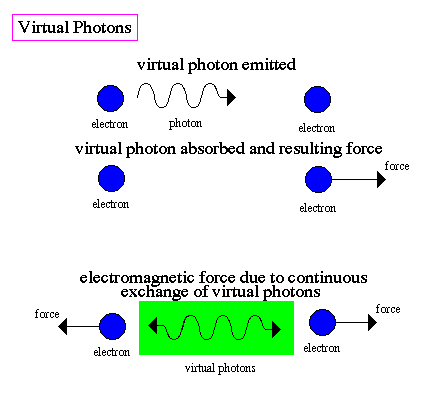.. QED led to the unification of electromagnetic and weak forces, implying that all forces are one force under extreme conditions of temperature and energy (like with the Universe formed) .. Notice the elimination of action at a distance, the interaction is due to direct contact of the photons. .. In the 1960's, a formulation of QED led to the unification of the theories of weak and electromagnetic interactions. This new force, called electroweak, occurs at extremely high temperatures such as those found in the early Universe and reproduced in particle accelerators. Unification means that the weak and electromagnetic forces become symmetric at this point, they behave as if they were one force. .. Electroweak unification gave rise to the belief that the weak, electromagnetic and strong forces can be unified into what is called the Standard Model of matter.

..
Quantum Chromodynamics:

 .. similar to QED, QCD describes the forces that bind quarks instead of virtual photons, the strong force is transfered by gluons each gluon can carry one of three color charges, red, blue or green so that particle built of quarks must be color neutral .. Quantum chromodynamics is the subfield of physics that describes the strong or "color" force that binds quarks together to form baryons and mesons, and results in the complicated force that binds atomic nuclei together. .. Quantum chromodynamics, or QCD, is the theory that describes the action of the strong nuclear force. QCD was constructed on analogy to quantum electrodynamics (QED), the quantum theory of the electromagnetic force. In QED, the electromagnetic interactions of charged particles are described through the emission and subsequent absorption of massless photons, best known as the "particles" of light; such interactions are not possible between uncharged, electrically neutral particles. The strong force is observed to behave in a similar way, acting only upon certain particles, principally quarks that are bound together in the protons and neutrons of the atomic nucleus, as well as in less stable, more exotic forms of matter. So by analogy with QED, quantum chromodynamics has been built upon the concept that quarks interact via the strong force because they carry a form of "strong charge," which has been given the name of color; other particles, such as the electron, which do not carry the color charge, do not interact in this way. .. In QED there are only two values for electric charge, positive and negative, or charge and anticharge. To explain the behavior of quarks in QCD, by contrast, there need to be three different types of color charge, each of which can occur as color or anticolor. The three types of charge are called red, green, and blue in analogy to the primary colors of light, although there is no connection whatsoever with color in the usual sense. .. Color-neutral particles occur in one of two ways. In baryons (i.e., particles built from three quarks, as, for example, protons and neutrons), the three quarks are each of a different color, and a mixture of the three colors produces a particle that is neutral. Mesons, on the other hand, are built from pairs of quarks and antiquarks, and in these the anticolor of the antiquark neutralizes the color of the quark, much as positive and negative electric charges cancel each other to produce an electrically neutral object.

..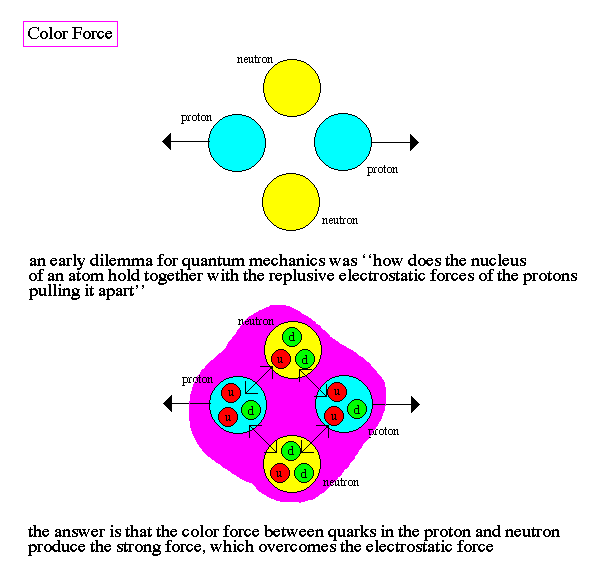.. note that the strong force overcomes the electromagnetic or gravitational forces only on very short range. Outside the nucleus the effect of the strong force is non-existent .. Quarks interact via the strong force by exchanging particles called gluons. In contrast to QED, where the photons exchanged are electrically neutral, the gluons of QCD also carry color charges. To allow all the possible interactions between the three colors of quarks, there must be eight gluons, each of which generally carries a mixture of a color and an anticolor of a different kind. .. Because gluons carry color, they can interact among themselves, and this makes the behavior of the strong force subtly different from the electromagnetic force. QED describes a force that becomes weaker as the distance between two charges increases (obeying an inverse square law), but in QCD the interactions between gluons emitted by color charges prevent those charges from being pulled apart. Instead, if sufficient energy is invested in the attempt to knock a quark out of a proton, for example, the result is the creation of a quark-antiquark pair--in other words a meson.

..
Quantum Gravity:

 Understanding of the fundamental forces of Nature will require a unification of quantum physics and general relativity the development of a quantum theory of spacetime, or quantum gravity, will begins with the discovery of the graviton, a quantum particle of spacetime Physicist frequently search for unifying principles that hopeful lead to deeper, more fundamental laws of Nature. The unification of the theory of electricity with the theory of magnetism led to an understanding of light as electromagnetic radiation. One obvious unification is between quantum mechanics and general relativity, the so-called theory of quantum gravity. .. Quantum gravity is a type of quantum theory of elementary particles and their interactions that is based on the particle symmetry known as supersymmetry and that naturally includes gravity along with the other fundamental forces (the electromagnetic force, the weak nuclear force, and the strong nuclear force). .. The electromagnetic and the weak forces are now understood to be different facets of a single underlying force that is described by the electroweak theory. Further unification of all four fundamental forces in a single quantum theory is a major goal of theoretical physics. Gravity, however, has proved difficult to treat with any quantum theory that describes the other forces in terms of messenger particles that are exchanged between interacting particles of matter. General relativity, which relates the gravitational force to the curvature of space-time, provides a respectable theory of gravity on a larger scale. To be consistent with general relativity, gravity at the quantum level must be carried by a particle, called the graviton.

..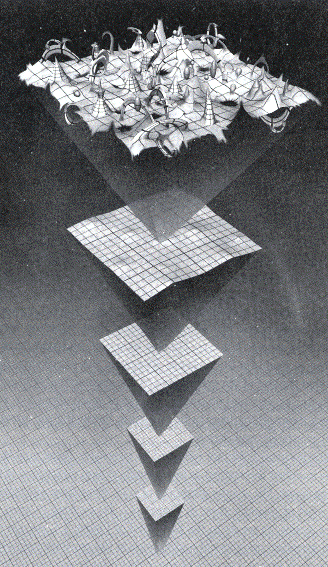.. exploring quantum gravity will require technology that is well beyond our current means .. Unifying theories usually begin by exploring new realms of experience. For example, particle theories (what are the fundamental particles that matter is made of) find deeper meaning by exploring high energies (i.e. high mass ranges) using large particle accelerators.

..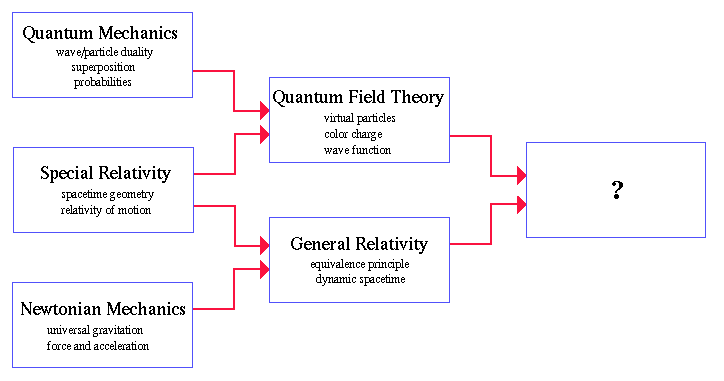.. theoretical work in quantum gravity asks questions about quantum sized black holes and a fuzzy event horizon .. Quantum gravity asks the question, "what is the behavior of gravity on extremely small scales?" What are the properties of mini black holes and how does the force of gravity compared to other subatomic forces? These questions are particularly crucial to cosmology since the very early Universe was an environment dominated by extremely high pressures and temperatures, and the folding of spacetime on quantum scales.

..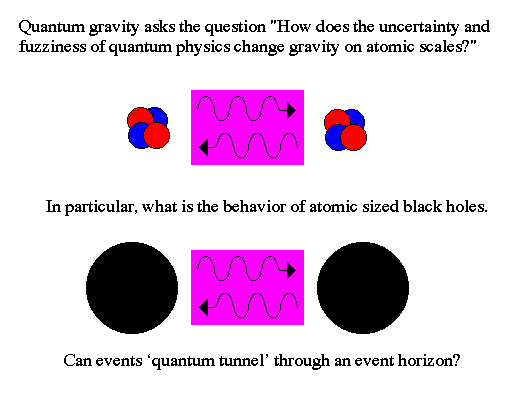.. while there is no current working quantum gravity theory, the path to TOE is through quantum gravity partial predictions from quantum gravity ideas indicates hope for a new direction in physics .. There is currently no complete theory for combined quantum and gravity, as the process of unification proved to have many more mathematical difficulties than expected. Many believe that the problems indicate that a new, much deeper theory must exist out of which quantum mechanics and general relativity emerge. However, some partial elements of a working composite of quantum mechanics and general relativity have predicted gravitational waves and Hawking radiation.

..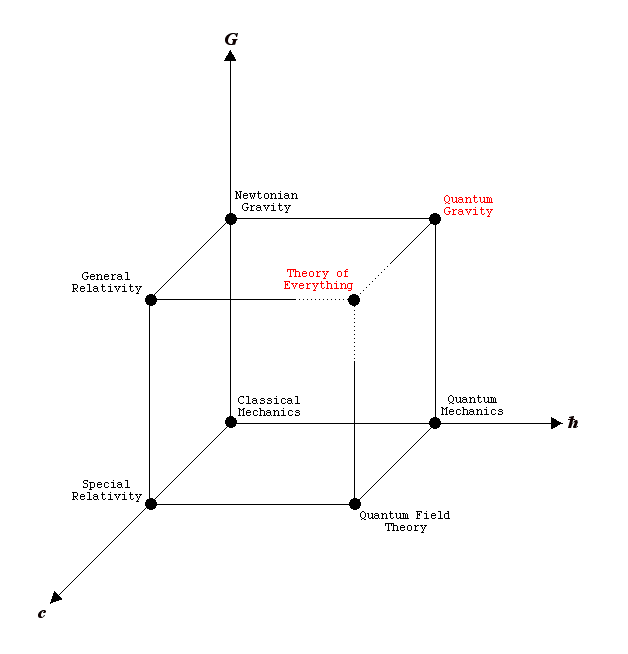..

 ripples in spacetime are gravity waves The existence of curved spacetime opens up the possibility that ripples or waves can exist in the spacetime continuum. These ripples are called gravitational waves. Gravity waves could be detected from colliding black holes, supernova explosions and the black hole at the core of our Galaxy.

.... to be generated, gravity waves require rapid motion of high density matter, like a supernova .. During the core collapse of the supernova, vast amounts of matter are moved about at enormous speeds. The dense mass is surrounded by a strong gravitational field. Vigorous changes in gravity will produce `ripples' in the geometry of space, and these ripples can propagate outward at the speed of light, i.e. gravity waves.

..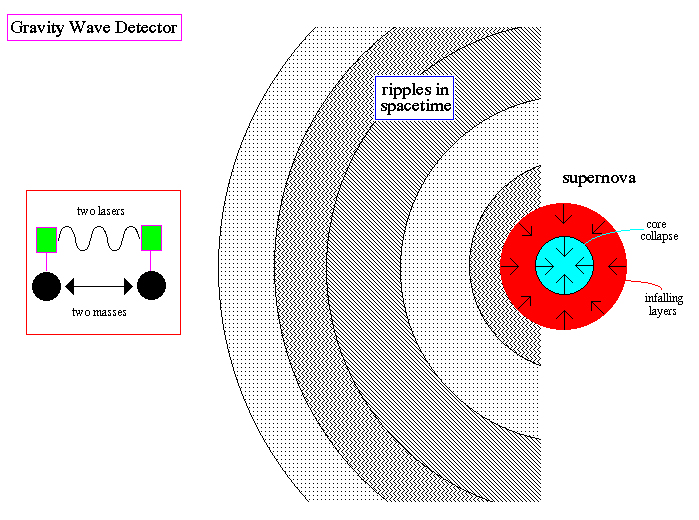.. gravity waves hope to be detected by new technology in this century .. Gravity waves can be detected by the effects they have on other masses. For example, two masses will vibrate when a gravity wave passes. Sensitive measurements of their motion with lasers will detect this motion. Currently our technology is unable to detect gravity waves, but a new laser system (called LIGO) is under construction for use in the 21st century. .. Gravitational wave astronomy could expand our knowledge of the cosmos dramatically. For starters, gravitational waves, though weakening with distance, are thought to be unchanged by any material they pass through and, therefore, should carry signals unaltered across the vast reaches of space. By comparison, electromagnetic radiation tends to be absorbed or modified by intervening matter.

..

 another example of quantum gravity phenomenon is Hawking radiation Hawking radiation explores the behavior of particle production near the event horizon of quantum sized black holes Hawking, an English theoretical physicist, was one of the first to consider the details of the behavior of a black hole whose Schwarzschild radius was on the level of an atom. These black holes are not necessarily low mass, for example, it requires 1 billion tons of matter to make a black hole the size of a proton. But their small size means that their behavior is a mix of quantum mechanics rather than relativity. .. Before black holes were discovered it was know that the collision of two photons can cause pair production. This a direct example of converting energy into mass (unlike fission or fusion which turn mass into energy). Pair production is one of the primary methods of forming matter in the early Universe.

..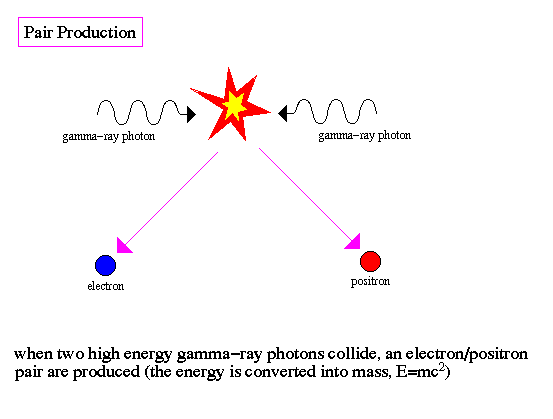.. since pair production is symmetric, matter and anti-matter are formed together energy from the black hole gravitational field is converted into matter .. Note that pair production is symmetric in that a matter and antimatter particle are produced (an electron and an anti-electron (positron) in the above example). .. Hawking showed that the strong gravitational gradients (tides) near black holes can also lead to pair production. In this case, the gravitational energy of the black hole is converted into particles.

..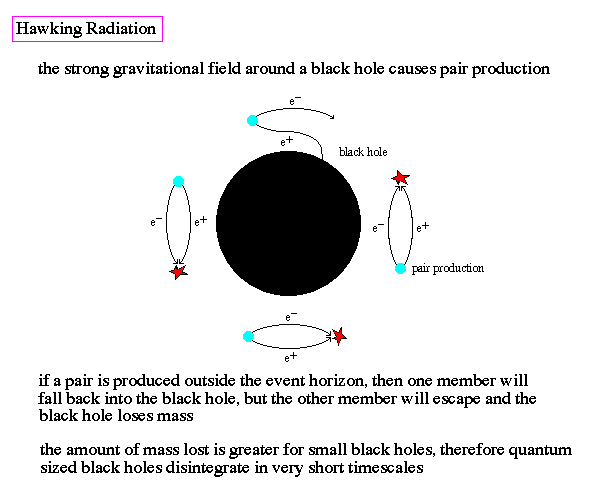.. Hawking radiation explains why there are no quantum sized black holes filling the Universe, they have dissolved into particles .. If the matter/anti-matter particle pair is produced below the event horizon, then particles remain trapped within the black hole. But, if the pair is produced above the event horizon, it is possible for one member to fall back into the black hole, the other to escape into space. Thus, the black hole can lose mass by a quantum mechanical process of pair production outside of the event horizon. The rate of pair production is stronger when the curvature of spacetime is high. Small black holes have high curvature, so the rate of pair production is inversely proportional to the mass of the black hole (this means its faster for smaller black holes). Thus, Hawking was able to show that the mini or primordial black holes expected to form in the early Universe have since disintegrated, resolving the dilemma of where all such mini-black holes are today.

..
Theory of Everything :

 the Standard model is our current theory of the matter/energy worldview, and has had great success with particle physics missing is a full formulation of gravity and particle physics, quantum gravity Is that it? Are quarks and leptons the fundamental building blocks? Answer = maybe. We are still looking to fill some holes in what is know as the Standard Model. .. The Standard Model is a way of making sense of the multiplicity of elementary particles and forces within a single scheme. The Standard Model is the combination of two schemes; the electroweak force (unification of electromagnetism and weak force) plus quantum chromodynamics. Although the Standard Model has brought a considerable amount of order to elementary particles and has led to important predictions, the model is not without some serious difficulties. .. For example, the problem of quantum gravity is unresolved in the Standard Model. Also undefined are the values of the various constants of Nature (the speed of light, charge on the electron, etc). In fact, why the Universe is built the way it is (3D+1D macroscopic world) is unclear, although various other dimensional shapes can be ruled out as shown by the diagram below.

..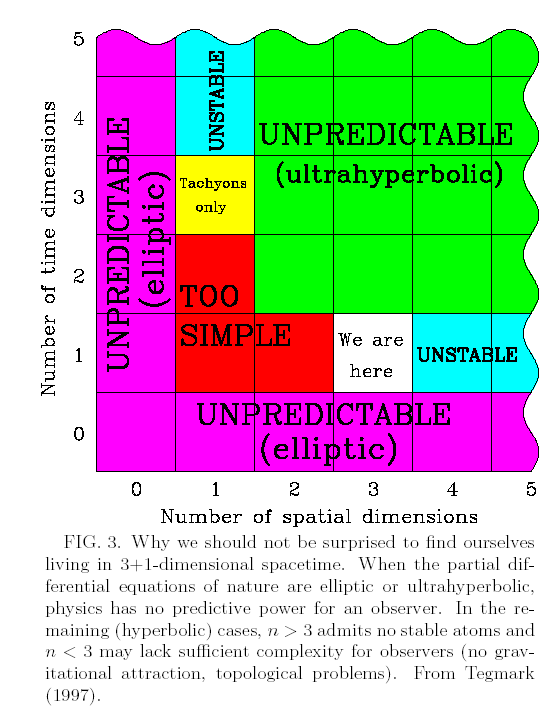.. limitations to the Standard Model suggests a more encompassing theory awaits formulation .. Another problem is that the Standard Model contains a large number of arbitrary constants. Good choice of the constants leads to exact matches with experimental results. However, a good fundamental theory should be one where the constants are self-evident. For example, the choice of 4 spacetime dimensions is understood under the Standard model since other choices are ruled out by mathematics. .. The Standard Model does not include the unification of all forces and, therefore, is incomplete. There is a strong expectation that there exists a Grand Unified Field Theory (GUTS) that will provide a deeper meaning to the Standard Model and explain the missing elements.

..
Supergravity:

 a theory that brings gravity, relativity and quantum physics together is called a Theory of Everything (TOE) one recent attempt is called supergravity, which explains the microscopic world as extra dimensions Even a GUTS is incomplete because it would not include spacetime and therefore gravity. It is hypothesized that a "Theory of Everything" (TOE) will bring together all the fundamental forces, matter and curved spacetime under one unifying picture. For cosmology, this will be the single force that controlled the Universe at the time of formation. The current approach to the search for a TOE is to attempt to uncover some fundamental symmetry, perhaps a symmetry of symmetries. There should be predictions from a TOE, such as the existence of the Higgs particle, the origin of mass in the Universe. .. One example of a attempt to formula a TOE is supergravity, a quantum theory that unities particle types through the use of ten dimensional spacetime (see diagram below). Spacetime (4D construct) was successful at explaining gravity. What if the subatomic world is also a geometric phenomenon. .. The idea that gravity is different from the other forces. While it is extremely long ranged, gravity is extremely weak (notice that a small magnet on the refrigerator can overcome the entire gravitational force of the Earth). Perhaps gravity is weak because it is `spread' out over many dimensions. This type of theory is called brane or membrane theory and hypothesizes that the 3D world is just one splice of a multi-dimensional space where gravity propagates between the branes (slices).

..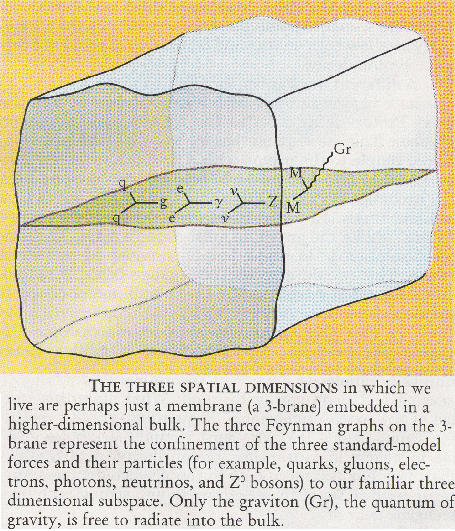.. using pure geometry is a popular feature to TOE's since they become fundamental in their mathematics as well as physics .. In fact, the brane theory doesn't necessary have to use stacks of other, `nearby' dimensions, the other dimensions could be small and `curled' into the microscopic world. Many more dimensions of time and space could lie buried at the quantum level, outside our normal experience, only having an impact on the microscopic world of elementary particles. .. It is entirely possible that beneath the quantum domain is a world of pure chaos, without any fixed laws or symmetries. One thing is obvious, that the more our efforts reach into the realm of fundamental laws, the more removed from experience are the results.

..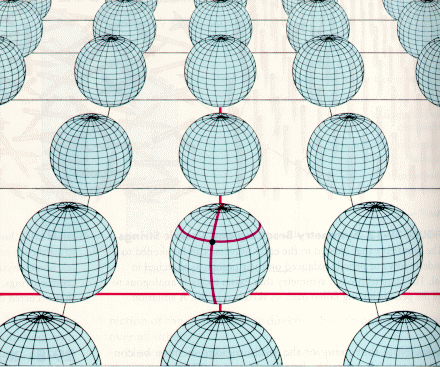..
String Theory:

 another example of a TOE is string theory, the explanation of quantum entities as tiny loops or membranes Another recent attempt to form a TOE is through M (for membrane) or string theory. String theory is actually a high order theory where other models, such as supergravity and quantum gravity, appear as approximations. The basic premise to string theory is that subatomic entities, such as quarks and forces, are actually tiny loops, strings and membranes that behave as particles at high energies.

..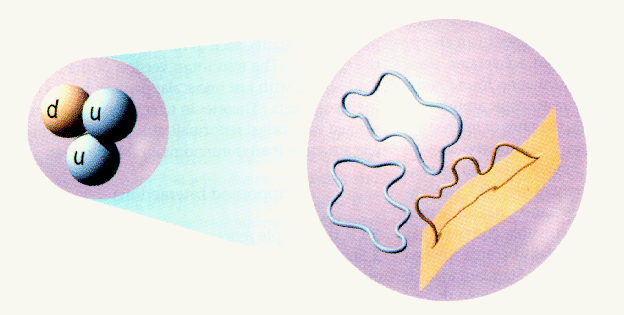.. the various subatomic particles are explained as different vibration modes of the tiny strings the rules for string interactions looks alot like spacetime and relativity .. One of the problems in particle physics is the bewildering number of elementary particles (muons and pions and mesons etc). String theory answers this problem by proposing that small loops, about 100 billion billion times smaller than the proton, are vibrating below the subatomic level and each mode of vibration represents a distinct resonance which corresponds to a particular particle. Thus, if we could magnify a quantum particle we would see a tiny vibrating string or loop. .. The fantastic aspect to string theory, that makes it such an attractive candidate for a TOE, is that it not only explains the nature of quantum particles but it also explains spacetime as well. Strings can break into smaller strings or combine to form larger strings. This complicated set of motions must obey self-consistent rules and the the constraint caused by these rules results in the same relations described by relativity theory. .. Another aspect of string theory that differs from other TOE candidates is its high aesthetic beauty. For string theory is a geometric theory, one that, like general relativity, describes objects and interactions through the use of geometry and does not suffer from infinites or what is called normalization problems such as quantum mechanics. It may be impossible to test the predictions of string theory since it would require temperature and energies similar to those at the beginning of the Universe. Thus, we resort to judging the merit of this theory on its elegance and internal consistence rather than experiment data.

..
Brane World Scenario:

 A combination of string theory and supergravity leads to an eleven dimensional description of the Universe called the brane world scenario Each brane universe is composed of 4D spacetime and 6D quantum space. The latest compulation of string theory and supergravity that brings together a multi-dimensional world view with the Standard Model is called brane world. In the brane world scenario, the entire Universe is an eleven dimensional `bulk' composed of an infinite number of ten dimensional branes. Each brane is consists of a macroscopic 4D spacetime and a compacted, microscopic 6D quantum world (a Calabi-Yau space). The Calabi-Yau sector is twisted beyond al possible detection and houses all the symmetries of the Standard model.

..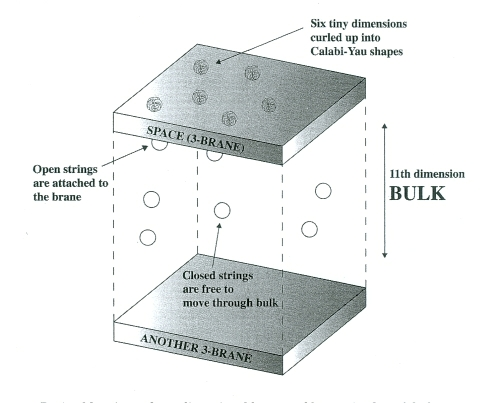.. Strong, weak and electromagnetic forces are carried by open strings, each attached to their branes Gravity is carried by closed strings, free to travel between branes .. The attractive aspect to the brane world scenario is that three of the four fundamental forces (strong, weak, electromagnetism and their assoicated particles) are represented by open strings. Gravity (and gravitons) are represented by closed strings (loops). Open strings are attached to their respective branes, but closed strings (gravity) are free to move between branes. This explains why gravity is so much weaker than the other forces, and gravity can be used to commiuncate between the branes (gravity phones). This also leads to a natural explanation for dark matter (see later lecture). .. All the branes are embedded in a higher dimension all the `bulk', a pile of parallel universes. Thus, the total Universe is eleven dimensional, 4D spacetime + 6D quantum space + 1D bulk. An infinite number of parallel universes is literally just a millimeter away, but outside your 4D vision.